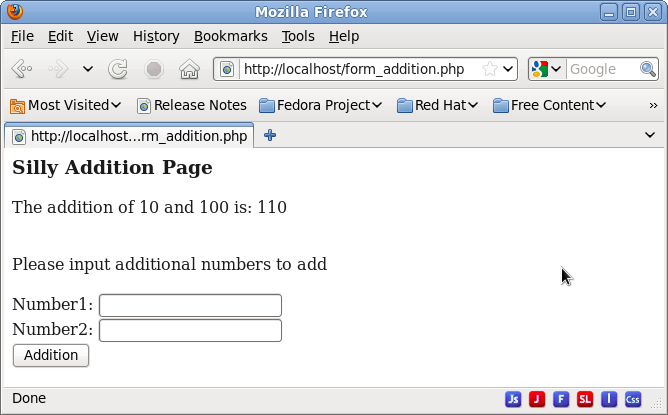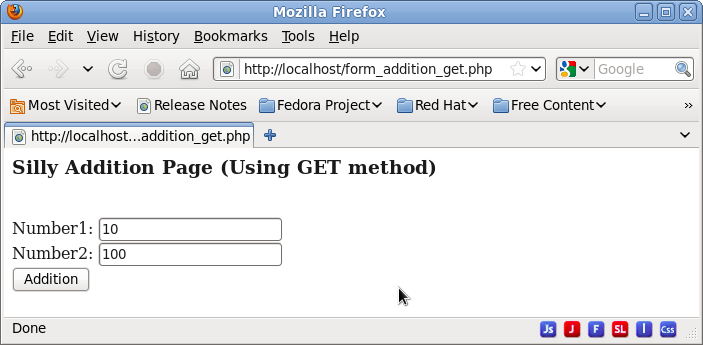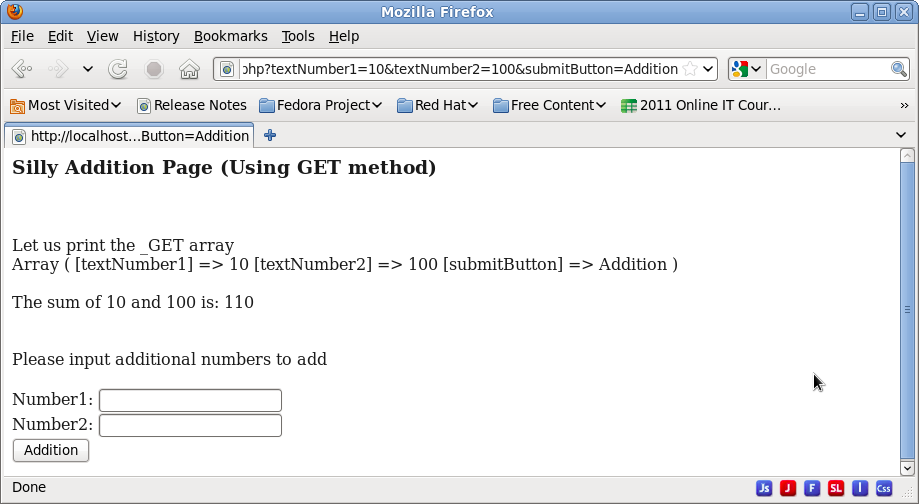## CodingBison

In HTML forms, the action files are typically different from the HTML form file. However, PHP does not prevent both the HTML form page and the action file to be the same file. Let us understand such self-referencing pages.

When using the same page for both form and its action file, the main requirement is to check if the page being displayed is called for the first time or is called after the user has submitted the form. The trick for this check is simple -- simply take a look at the \$_POST array; if \$_POST array is NULL, then this page is being loaded for the first time, otherwise, we are here because of self-reference.

Here is the flow:

``` 			      --------------------------->
^	 		         |
HTML Form     |			         |
---------------------- 		         | Submit the Form. Form data
|"form_addition.php" |                   | is passed as \$_POST array.
----------------------	                 |
^ Form Action File	 |
|				 V
<--------------------------

Figure: HTML Flow when submitting a Form. Both HTML Form and Form Action file
reside in the same file.
```

We present a simple PHP example that has a form along with the PHP logic to handle input from the same form (we call it "form_addition.php"). As shown in the above figure, when we submit the form, we get forwarded to the same file again.

For "form_addition.php" (provided below), if the user enters the two variables and submits the form, then the same page is revisited and the "if (\$_POST)" condition becomes true. Since we know that we are here with some input (since \$_POST is not NULL), we add the two input. Next, we re-display the form so that the user can add additional variables.

``` <!doctype html>
<html>

<body>
<?php
if (\$_POST) {

if (is_numeric(\$_POST["textNumber1"]) and
is_numeric(\$_POST["textNumber2"]) ) {
/* Add if inputs are numeric */
} else {
/* Concatenate if inputs are non-numeric */
}

}
?>

<form name="input_form" method="POST" action="<?php echo \$_SERVER['PHP_SELF'] ?>">
<br> Number1: <input type="text" name="textNumber1">
<br> Number2: <input type="text" name="textNumber2">
</form>

</body></html>
```

Here is the output of this form, before and after clicking the "Addition" button. As we can see, after clicking the button, the same file displays the output of the earlier input and then re-displays the form.Figure: Form before clicking "Addition" buttonFigure: Form after clicking "Addition" button

### Using self-referencing forms with GET Method

All the earlier examples have used the "POST" method when submitting the form. However, we can also use "GET" method to do the same. Let us begin by rewriting the above example of self-referencing page to use "GET" method instead of "POST"; we name this file "form_addition_get.php".

``` <!doctype html>
<html>

<?php
if (\$_GET) {

echo "<br><br>Let us print the _GET array <br>";
print_r(\$_GET);

if (is_numeric(\$_GET["textNumber1"]) and
is_numeric(\$_GET["textNumber2"]) ) {
/* Add if inputs are numeric */
} else {
/* Concatenate if inputs are non-numeric */
}

}
?>

<body>
<form name="input_form" method="GET" action="<?php echo \$_SERVER['PHP_SELF'] ?>">
<br> Number1: <input type="text" name="textNumber1">
<br> Number2: <input type="text" name="textNumber2">
</form>

</body></html>
```

As we can see, in terms of form elements and its processing on the action file, they both act similarly. The \$_GET array has same elements as that of \$_POST array in the previous example; \$_GET is an alias for \$HTTP_GET_VARS array. This commonality is because when we submit the button, both of these methods (POST and GET) pass the form data to the action file.Figure: Form before clicking "Addition" buttonFigure: Form after clicking "Addition" button

However, the main difference between these two methods surfaces when we observe the URL after hitting the submit button. With GET method, the form elements and their values get encoded with the URL and thus, are visible on the browser address when we hit the submit button. Thus, when we enter values like 10 and 100 for the two numbers, then the URL becomes "http://localhost/form_addition_get.php?textNumber1=10&textNumber2=100&submitButton=Addition". With "POST" method, all we saw was: "http://localhost/form_addition_get.php".

In fact, for the "GET" method, we could just easily modify these values in the browser address bar and hit enter. Thus, we do not have to depend upon taking the input from the form at all!